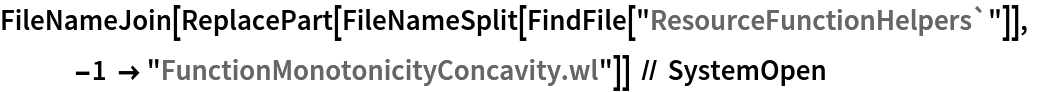#Function Repository Resource:

# FunctionConcavity (1.0.0)current version: 2.0.0 »

Compute the regions on which an expression is concave up or down

Contributed by: Wolfram|Alpha Math Team
 ResourceFunction["FunctionConcavity"][f,x] returns an association of information about whether f is concave up or concave down with respect to x. ResourceFunction["FunctionConcavity"][f,x,property] returns a specific property related to whether f is concave up or concave down with respect to x.

## Details and Options

ResourceFunction["FunctionConcavity"] expects f to be a univariate expression in terms of x, similar to what might be entered into Plot.
ResourceFunction["FunctionConcavity"] returns regions on which the second derivative of expr with respect to x is greater than 0 (concave up) or less than 0 (concave down).
The input property can be any of All, "ConcaveUp", "ConcaveDown", "Regions", "Plot" or "NumberLine", and defaults to "Regions".

## Examples

### Basic Examples (2)

Compute the regions on which a curve is concave up or down:

 In:=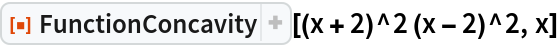Out=Return plots as well as the regions:

 In:=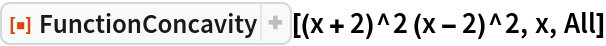Out=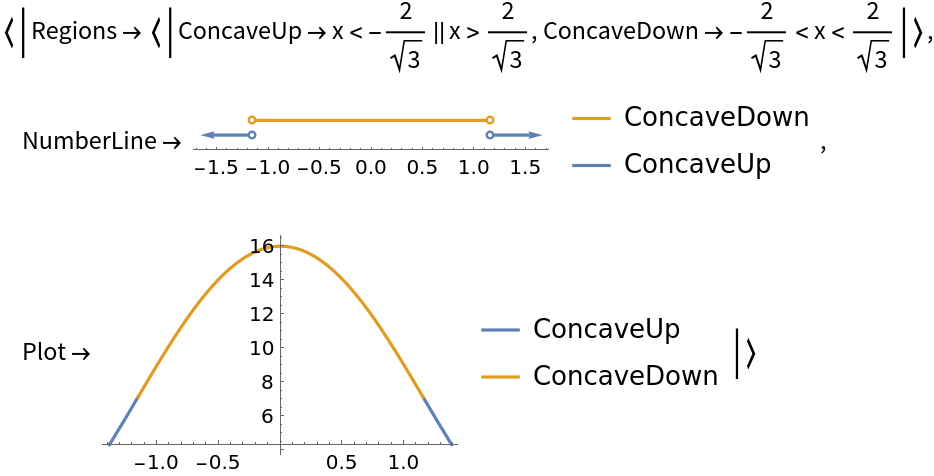### Scope (4)

Use the "NumberLine" property to visualize the regions directly:

 In:=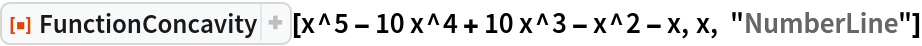Out=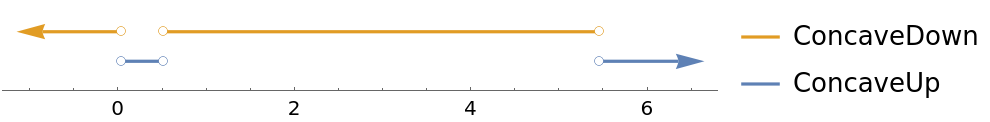Use the "Plot" property to visualize the regions on the plot of the curve:

 In:=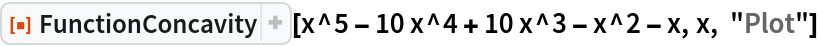Out=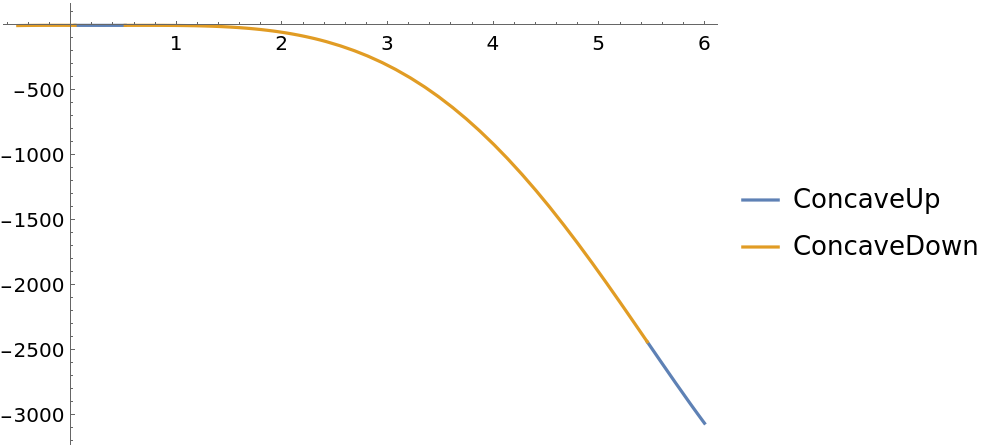Note that at stationary points of the expression, the curve is neither concave up nor concave down. In this case, 0 is a member of neither of the regions:

 In:=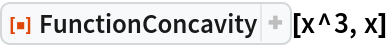Out=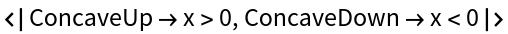To test that 0 is the only point where the second derivative is 0, use Resolve:

 In:=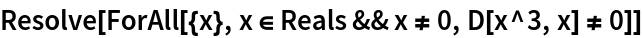Out=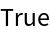FunctionConcavity returns strict regions of the second derivative being non-zero as can be seen in this Piecewise expression:

 In:=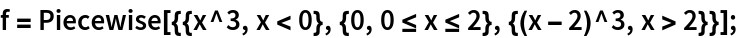In:=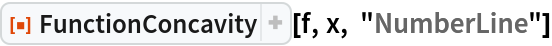Out=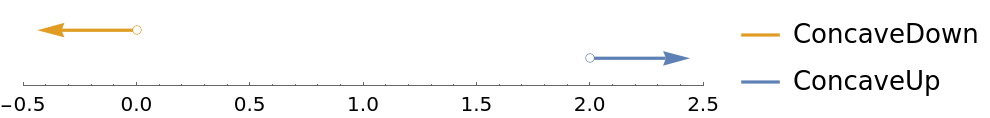In:=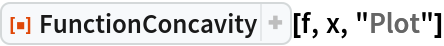Out=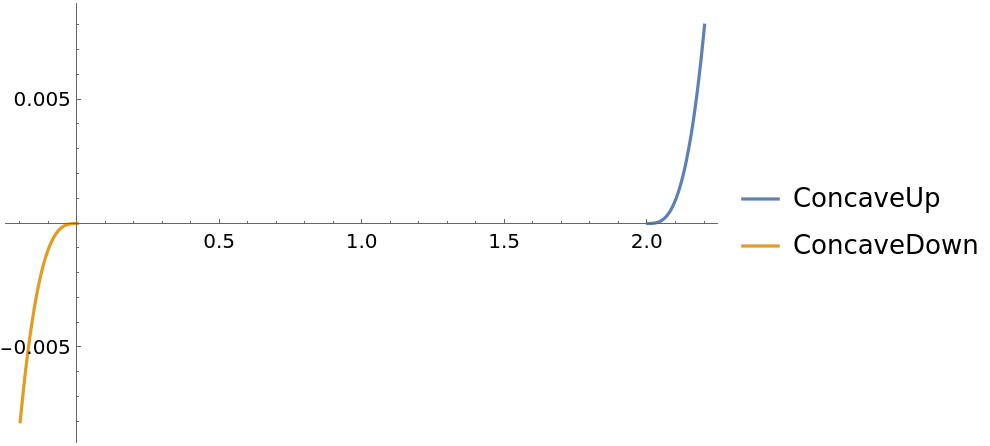### Possible Issues (3)

Working with the absolute value function Abs may return unexpected results:

 In:=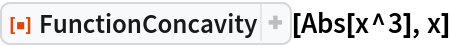Out=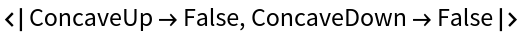This is due to the assumption of Complex inputs to Abs in comparison to RealAbs:

 In:=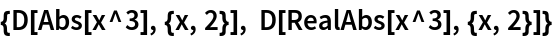Out=Using RealAbs returns the expected results:

 In:=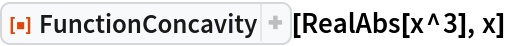Out=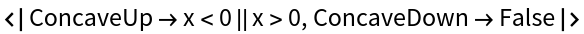### Neat Examples (1)

Compute the concavity of a periodic function and visualize it:

 In:=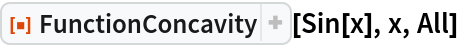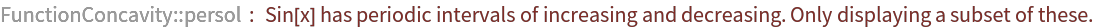Out=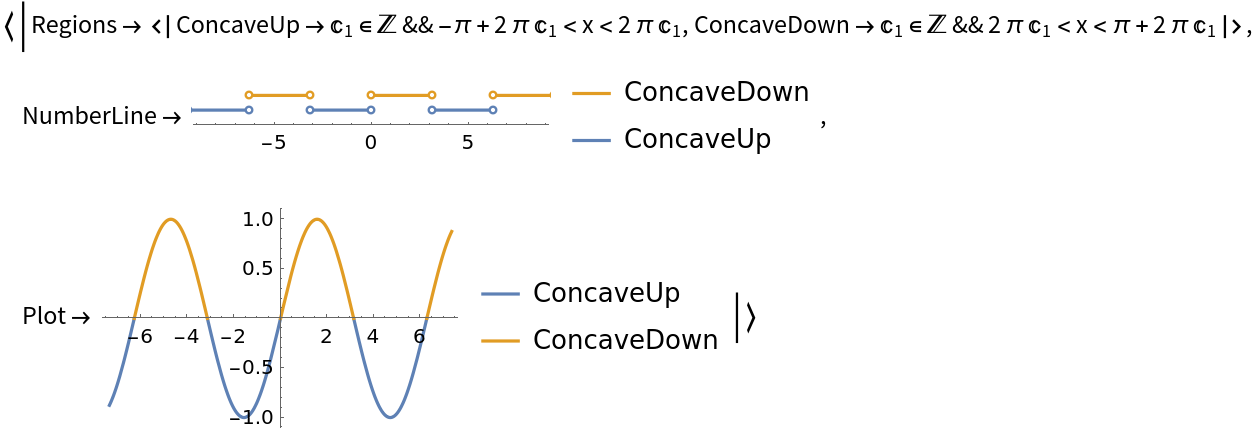## Publisher

Wolfram|Alpha Math Team

## Version History

• 2.0.0 – 23 March 2023
• 1.0.0 – 18 September 2020

## Author Notes

To view the full source code for FunctionConcavity, run the following code: